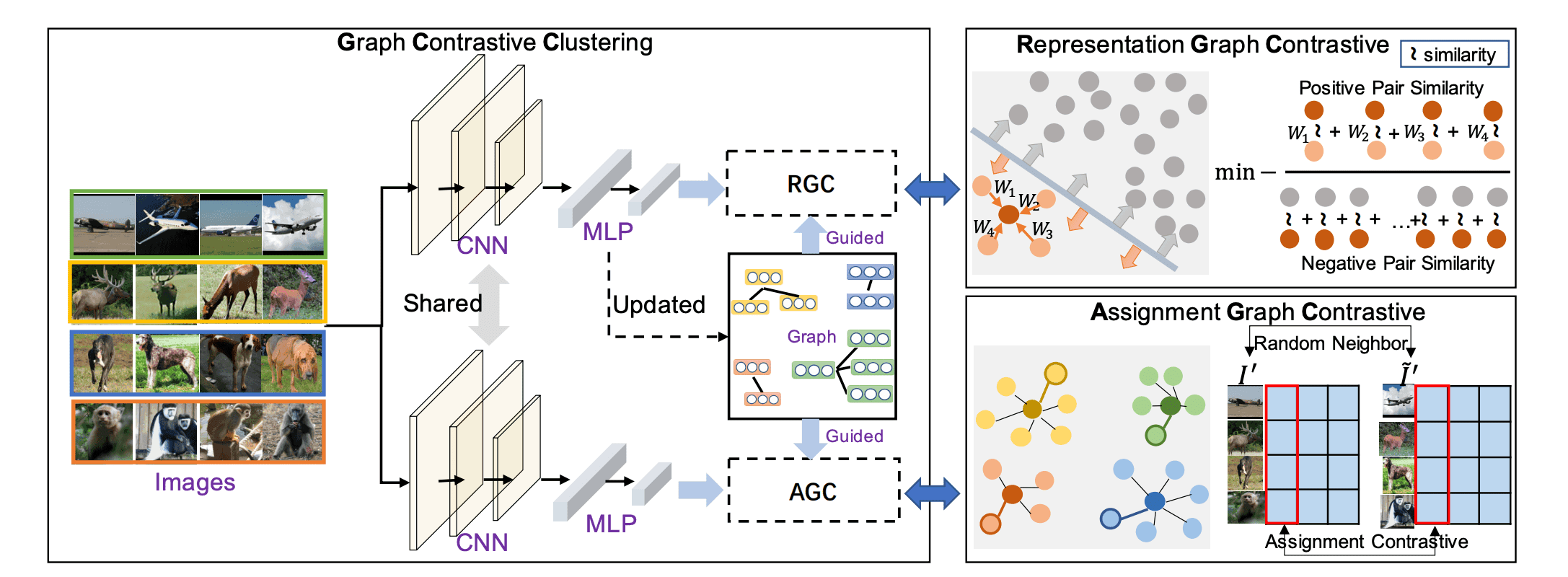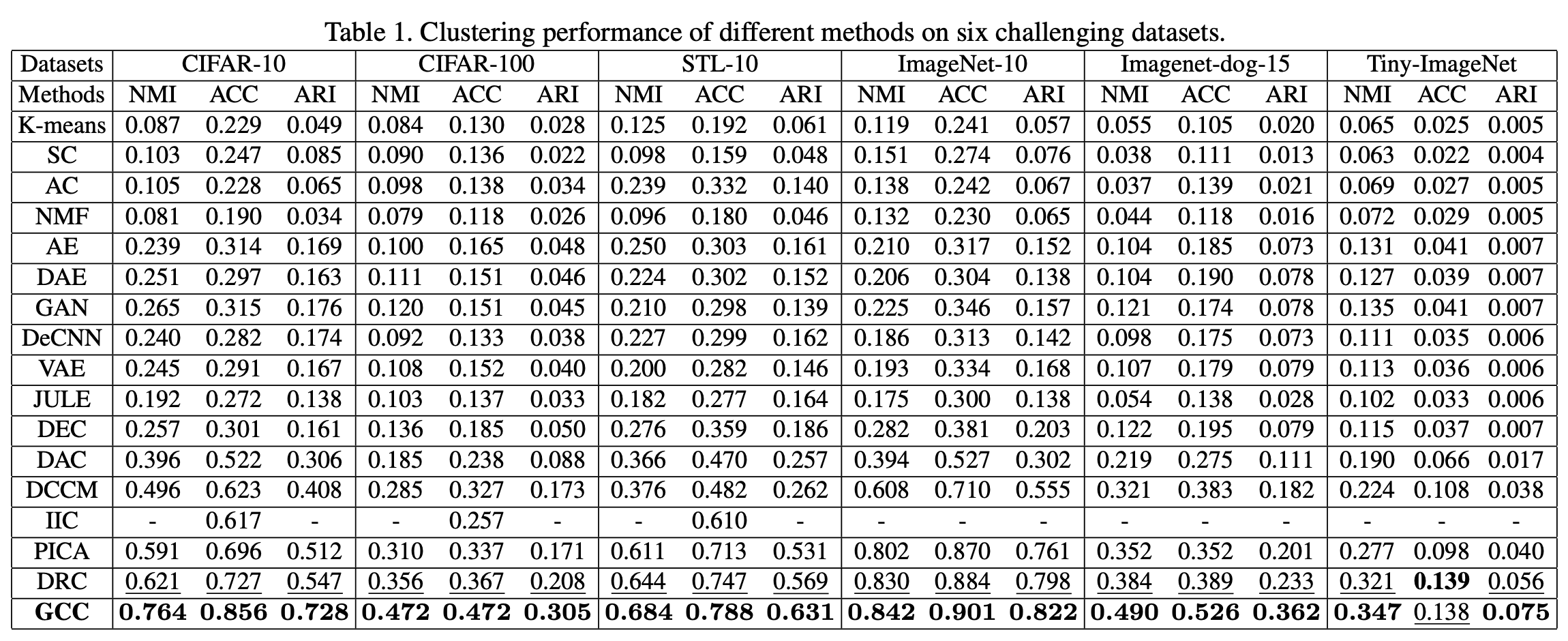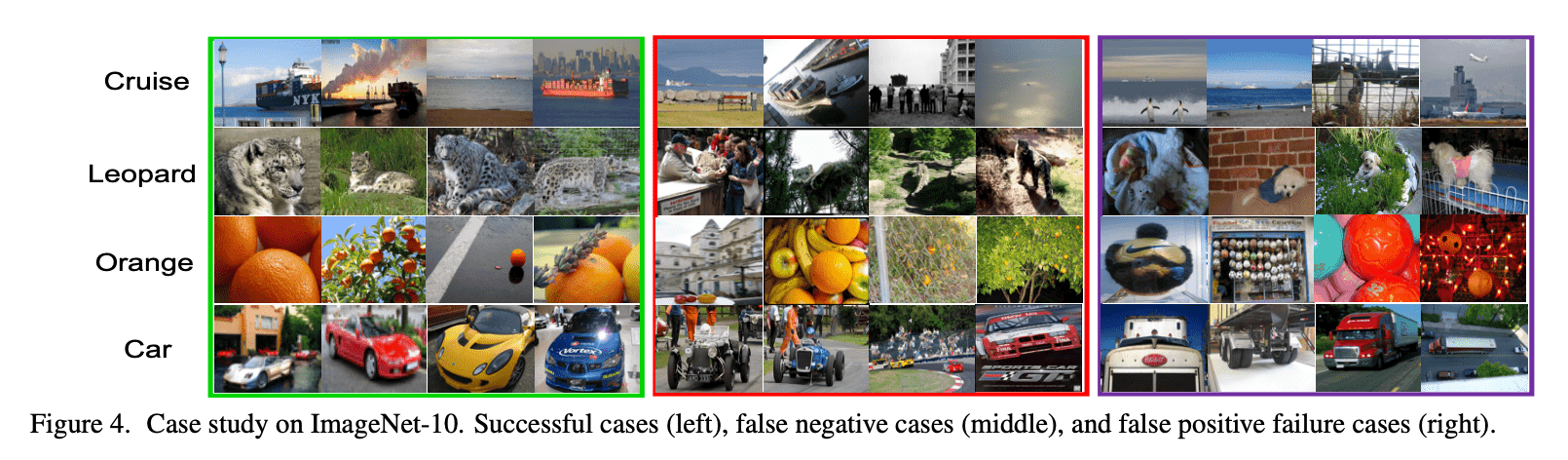# Catch up on the latest AI articles# Graph Contrastive Clustering

3 main points
✔️ We developed a novel graph contrastive framework that learns to ensure that samples in the same cluster and augmentation results have a similar amount of representations.
✔️ We applied the above framework to clustering and introduced a module for learning features with high discriminative performance and a module for more compact cluster assignment.
✔️ image clustering experiments on a variety of datasets. and recorded significantly higher accuracy than existing models.

written by Huasong ZhongJianlong WuChong ChenJianqiang HuangMinghua DengLiqiang NieZhouchen LinXian-Sheng Hua
(Submitted on 3 Apr 2021)

Subjects:  Computer Vision and Pattern Recognition (cs.CV)code：The images used in this article are from the paper, the introductory slides, or were created based on them.

## first of all

Recently, contrastive learning, which simultaneously performs representation amount and clustering assignment, has been proposed. However, the clustering may be limited by the fact that conventional methods do not consider categorical information. Therefore, in this paper, we developed a new graph contrastive framework and proposed graph contrastive clustering (GCC) applied to clustering.

## technique

$N$ unlabeled $N$ images belonging to $K$ kinds of categories Given a set of images ${\bf I}=\{I_1,I_2,\cdots,I_N\}$, clustering aims to classify them into $K$ kinds of clusters. To obtain the features, we train a CNN model $\Phi(\theta)$ and map $I_i$ to $(z_i,p_i)$, which are $d$-dimensional feature representations ($||z_i|||_2=1$) and $K$-dimensional cluster assignment probability distributions ($\sum_{j=1}^Kp_{ij}= 1$). The predicted labels for the $i$-th sample are obtained as follows

$$l_i = {\rm arg max}_j(p_{ij}), \ 1\le j \le K$$

### Graph Contrastive (GC)

Let $G=(V,E)$ be an undirected graph with vertex $V=\{v_1,\cdots,v_N\}$ and edges $E$. Let $G=(V,E)$ be an undirected graph with vertex $V=\{v_1,\cdots,v_N\}$ and edge $E$ and define the adjacency matrix $A$ as

$$A_{ij}=\begin{cases}1, & {\rm if}(v_i, v_j)\in E \\0, & {\rm otherwise} \end{cases}$$

Let $D_i$ be the order of $V_i$ and the matrix $D$.

$$d_{ij}=\begin{cases}d_i, & (i=j) \\0, & (i\neq j) \end{cases}$$

be a matrix with $ij$ components, the normalized symmetric graph Laplacian is defined to be

$$L=I-D^{-\frac{1}{2}}AD^{-\frac{1}{2}},\ L_{ij}=-\frac{A_{ij}}{\sqrt{d_id_j}}(i\neq j)$$

Given $N$ normalized $N$ feature representations ${\bf x}=\{x_1,\cdots, x_N\}$, GC makes $x_i$ and $x_j$ closer if $A_{ij}>0$ and $x_i$ and $x_j$ farther if $A_{ij}=0$. If the graph is divided into multiple communities, then the similarity of feature representations of the same community is greater than that of different communities. Define the similarity $S_{intra}$ and the similarity $S_{inter}$ of the inner and outer communities, respectively, as follows.

$$S_{intra}=\sum_{L_{ij}<0}-L_{ij}S(x_i,x_j)$$

$$S_{inter}=\sum_{L_{ij}=0}S(x_i,x_j)$$

However $S(x_i,x_j)$ is the similarity and in this paper we used Gaussian kernel.

$$S(x_i,x_j)=e^{-||x_i-x_j||_2^2/\tau}\sim e^{x_i\cdot x_j/\tau}$$

Thus the GC loss is

$${\cal L}_{GC}=-\frac{1}{N}\sum_{i=1}^N\log(\frac{S_{intra}}{S_{inter}})$$

is the same as the

### GCC

The GCC framework is outlined in the figure below.It consists of two heads that share a CNN and consists of a Representation Graph Contrastive (RGC) module that learns features and an Assignment Graph Contrastive (AGC) module that learns cluster assignments.

### RGC

Let ${\bf I}'=\{I_1',\cdots,I_N'\}$ be a random transformation of the original image and $z'=(z_1',\cdots,z_N')$ their features, the RGC loss can be written from the above discussion as follows.

$$L_{RGC}^{(t)}=-\frac{1}{N}\sum_{i=1}^N\log\left(\frac{\sum_{L_{ij}^{(t)}<0}-L_{ij}^{(t)}e^{z_i'\cdot z_j'/\tau}}{\sum_{L_{ij}=0}e^{z_i'\cdot z_j'/\tau}}\right)$$

### AGC

Let ${\bf {\tilde I}}'=\{{\tilde I}_1',\cdots,{\tilde I}_N'\}$ be a vector such that ${\tilde I}_j'$ is a randomly transformed image of a random neighborhood sample of $I_i$ based on $A^{(t)}$. Each probability vector is

$${\bf q}'=[q_1',\cdots, q_K']_{N\times K}$$

$${\tilde {\bf q}}'=[{\tilde q}_1',\cdots,{\tilde q}_K']_{N\times K}$$

We can express this as where $q_i'$ and ${\tilde q}_i'$ are the probability vectors of which samples in ${\bf I}'$ and ${\tilde {\bf I}}'$ belong to cluster $i$, respectively.

$${\cal L}_{AGC}=-\frac{1}{K}\sum_{i=1}^K\log\left(\frac{e^{q_i'\cdot q_i'/\tau}}{\sum_{j=1}^Ke^{q_i'\cdot q_j'/\tau}}\right)$$

### Loss function

We also add the following regularization loss to avoid a locally optimal solution.

$${\cal L}_{CR}=\log(K)-H(\cal Z)$$

However, $H$ is entropy, ${\cal Z}_i=\frac{\sum_{j=1}^Nq_{ij}}{\sum_{i=1}^K\sum_{j=1}^Nq_{ij}}$. The final loss function is

$${\cal L}={\cal L}_{RGC}+\lambda{\cal L}_{AGC}+\eta{\cal L}_{CR}$$

The result is as follows. where $\lambda, \eta$ is a hyperparameter.

## result

We compared the performance with traditional methods on six different benchmark datasets. The results are shown in the table below.From the table, it can be seen that the method in this paper is effective in all three evaluation metrics, as it significantly improves the traditional SOTA model for all data sets. Examples of successful and unsuccessful GCC samples are also shown in the figure below.From the figure, the successful samples (left) include those with different backgrounds and angles, showing robustness. On the other hand, the unsuccessful samples (center, right) include other classes with similar shapes or those with different patterns, and it is difficult to separate them in unsupervised learning, which is a challenge.

## summary

In this paper, we proposed a new graph Contrastive learning-based clustering method. Unlike conventional methods, we learned efficiently by bringing the similarity of the augmentations of a sample and other samples belonging to the same cluster closer together. As a result, we significantly outperformed the conventional SOTA on six benchmarks.

If you have any suggestions for improvement of the content of the article,